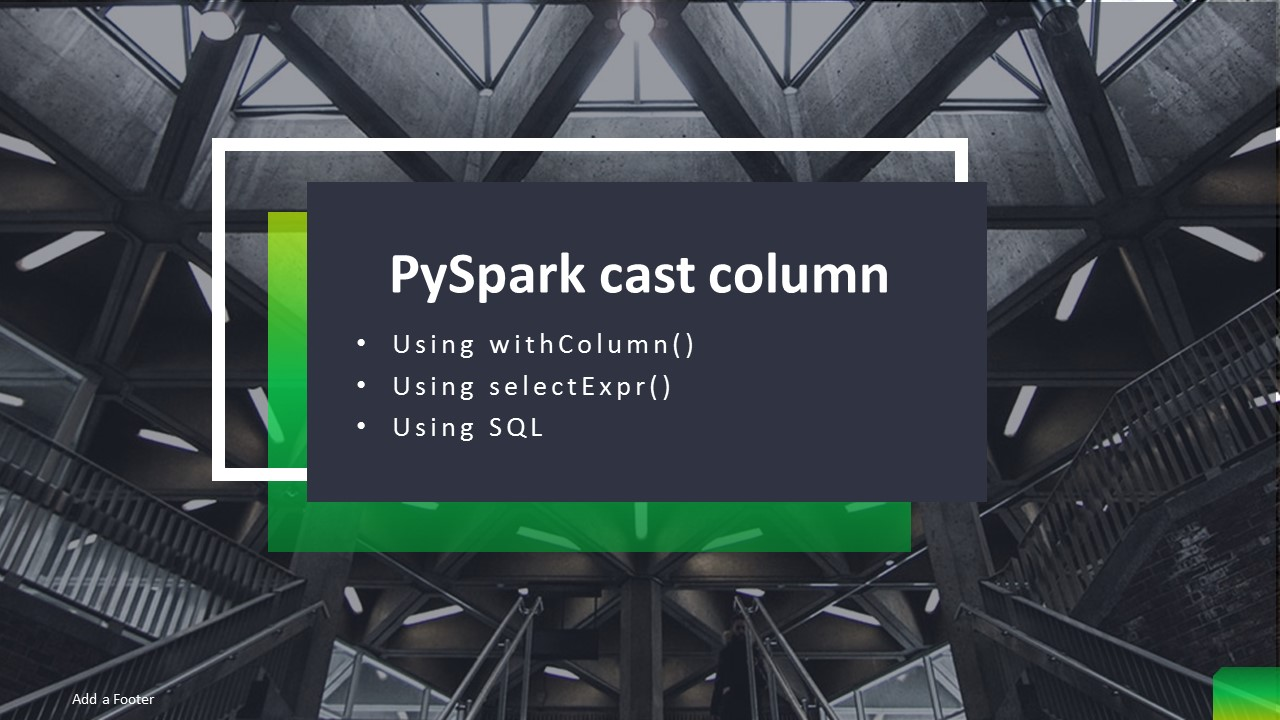# PySpark – Cast Column Type With Examples

In PySpark, you can cast or change the DataFrame column data type using `cast()` function of `Column` class, in this article, I will be using withColumn(), `selectExpr()`, and SQL expression to cast the from String to Int (Integer Type), String to Boolean e.t.c using PySpark examples.

Note that the type which you want to convert to should be a subclass of DataType class.

Below are the subclasses of the DataType classes in PySpark and we can change or cast DataFrame columns to only these types.

## 1. Cast Column Type With Example

Below are some examples that convert String Type to Integer Type (int)

``````
from pyspark.sql.types import IntegerType,BooleanType,DateType
# Convert String to Integer Type
df.withColumn("age",df.age.cast(IntegerType()))
df.withColumn("age",df.age.cast('int'))
df.withColumn("age",df.age.cast('integer'))

# Using select
df.select(col("age").cast('int').alias("age"))

#Using selectExpr()
df.selectExpr("cast(age as int) age")

#Using with spark.sql()
``````

Let’s run with an example, first, create simple DataFrame with different data types.

``````
import pyspark
from pyspark.sql import SparkSession

spark = SparkSession.builder.appName('SparkByExamples.com') \
.getOrCreate()

simpleData = [("James",34,"2006-01-01","true","M",3000.60),
("Michael",33,"1980-01-10","true","F",3300.80),
("Robert",37,"06-01-1992","false","M",5000.50)
]

df = spark.createDataFrame(data = simpleData, schema = columns)
df.show(truncate=False)
``````

Outputs:

``````
root
|-- firstname: string (nullable = true)
|-- age: long (nullable = true)
|-- jobStartDate: string (nullable = true)
|-- isGraduated: string (nullable = true)
|-- gender: string (nullable = true)
|-- salary: double (nullable = true)

+---------+---+------------+-----------+------+------+
+---------+---+------------+-----------+------+------+
|James    |34 |2006-01-01  |true       |M     |3000.6|
|Michael  |33 |1980-01-10  |true       |F     |3300.8|
|Robert   |37 |06-01-1992  |false      |M     |5000.5|
+---------+---+------------+-----------+------+------+
``````

## 2. withColumn() – Change Column Type

Use withColumn() to convert the data type of a DataFrame column, This function takes column name you wanted to convert as a first argument and for the second argument apply the casting method `cast()` with DataType on the column.

Below PySpark, snippet changes DataFrame column, `age` from Integer to String (StringType), isGraduated column from String to Boolean (BooleanType) and jobStartDate column to Convert from String to DateType.

``````
from pyspark.sql.functions import col
from pyspark.sql.types import StringType,BooleanType,DateType
df2 = df.withColumn("age",col("age").cast(StringType())) \
.withColumn("jobStartDate",col("jobStartDate").cast(DateType()))
df2.printSchema()
``````

Outputs:

``````
root
|-- firstname: string (nullable = true)
|-- age: string (nullable = true)
|-- jobStartDate: date (nullable = true)
|-- isGraduated: boolean (nullable = true)
|-- gender: string (nullable = true)
|-- salary: double (nullable = true)
``````

## 3. selectExpr() – Change Column Type

`selectExpr()` is a function in DataFrame which we can use to convert spark DataFrame column “age” from String to integer, “isGraduated” from boolean to string and “jobStartDate” from date to String.

``````
df3 = df2.selectExpr("cast(age as int) age",
"cast(jobStartDate as string) jobStartDate")
df3.printSchema()
df3.show(truncate=False)
``````
``````
root
|-- age: integer (nullable = true)
|-- isGraduated: string (nullable = true)
|-- jobStartDate: string (nullable = true)

+---+-----------+------------+
+---+-----------+------------+
|34 |true       |2006-01-01  |
|33 |true       |1980-01-10  |
|37 |false      |null        |
+---+-----------+------------+
``````

## 4. SQL – Cast using SQL expression

We can also use PySpark SQL expression to change/cast the spark DataFrame column type. In order to use on SQL, first, we need to create a table using `createOrReplaceTempView()`. On SQL just wrap the column with the desired type you want.

``````
df3.createOrReplaceTempView("CastExample")
df4 = spark.sql("SELECT STRING(age),BOOLEAN(isGraduated),DATE(jobStartDate) from CastExample")
df4.printSchema()
df4.show(truncate=False)
``````

This yields the below output.

``````
root
|-- age: string (nullable = true)
|-- isGraduated: boolean (nullable = true)
|-- jobStartDate: date (nullable = true)

``````

## 5. Complete Example of Casting PySpark Column

Below is complete working example of how to convert the data types of DataFrame column.

``````
import pyspark
from pyspark.sql import SparkSession

spark = SparkSession.builder.appName('SparkByExamples.com').getOrCreate()

simpleData = [("James",34,"2006-01-01","true","M",3000.60),
("Michael",33,"1980-01-10","true","F",3300.80),
("Robert",37,"06-01-1992","false","M",5000.50)
]

df = spark.createDataFrame(data = simpleData, schema = columns)
df.printSchema()
df.show(truncate=False)

from pyspark.sql.functions import col
from pyspark.sql.types import StringType,BooleanType,DateType

df2 = df.withColumn("age",col("age").cast(StringType())) \
.withColumn("jobStartDate",col("jobStartDate").cast(DateType()))
df2.printSchema()

df3 = df2.selectExpr("cast(age as int) age",
"cast(jobStartDate as string) jobStartDate")
df3.printSchema()
df3.show(truncate=False)

df3.createOrReplaceTempView("CastExample")
df4 = spark.sql("SELECT STRING(age),BOOLEAN(isGraduated),DATE(jobStartDate) from CastExample")
df4.printSchema()
df4.show(truncate=False)
``````

This example is also available at GitHub for reference.

## 6. Conclusion

In this PySpark article, you have learned how to cast or change one DataFrame column Data Type to another type using `withColumn()`, `selectExpr()`, SQL.

Happy Learning !!

### NNK

SparkByExamples.com is a Big Data and Spark examples community page, all examples are simple and easy to understand and well tested in our development environment Read more ..